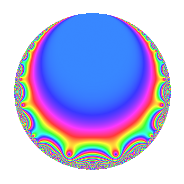# Properties

 Label 1155.2.a.qLevel 1155 Weight 2 Character orbit 1155.a Self dual Yes Analytic conductor 9.223 Analytic rank 0 Dimension 2 CM No Inner twists 1

# Learn more about

## Newspace parameters

 Level: $$N$$ = $$1155 = 3 \cdot 5 \cdot 7 \cdot 11$$ Weight: $$k$$ = $$2$$ Character orbit: $$[\chi]$$ = 1155.a (trivial)

## Newform invariants

 Self dual: Yes Analytic conductor: $$9.22272143346$$ Analytic rank: $$0$$ Dimension: $$2$$ Coefficient field: $$\Q(\sqrt{6})$$ Coefficient ring: $$\Z[a_1, a_2]$$ Coefficient ring index: $$1$$ Fricke sign: $$-1$$ Sato-Tate group: $\mathrm{SU}(2)$

## $q$-expansion

Coefficients of the $$q$$-expansion are expressed in terms of $$\beta = \sqrt{6}$$. We also show the integral $$q$$-expansion of the trace form.

 $$f(q)$$ $$=$$ $$q$$ $$+ \beta q^{2}$$ $$+ q^{3}$$ $$+ 4 q^{4}$$ $$+ q^{5}$$ $$+ \beta q^{6}$$ $$+ q^{7}$$ $$+ 2 \beta q^{8}$$ $$+ q^{9}$$ $$+O(q^{10})$$ $$q$$ $$+ \beta q^{2}$$ $$+ q^{3}$$ $$+ 4 q^{4}$$ $$+ q^{5}$$ $$+ \beta q^{6}$$ $$+ q^{7}$$ $$+ 2 \beta q^{8}$$ $$+ q^{9}$$ $$+ \beta q^{10}$$ $$- q^{11}$$ $$+ 4 q^{12}$$ $$+ ( 2 - \beta ) q^{13}$$ $$+ \beta q^{14}$$ $$+ q^{15}$$ $$+ 4 q^{16}$$ $$-3 q^{17}$$ $$+ \beta q^{18}$$ $$- q^{19}$$ $$+ 4 q^{20}$$ $$+ q^{21}$$ $$-\beta q^{22}$$ $$+ ( 3 - 2 \beta ) q^{23}$$ $$+ 2 \beta q^{24}$$ $$+ q^{25}$$ $$+ ( -6 + 2 \beta ) q^{26}$$ $$+ q^{27}$$ $$+ 4 q^{28}$$ $$+ ( -3 + \beta ) q^{29}$$ $$+ \beta q^{30}$$ $$+ ( 2 - 3 \beta ) q^{31}$$ $$- q^{33}$$ $$-3 \beta q^{34}$$ $$+ q^{35}$$ $$+ 4 q^{36}$$ $$+ ( 2 + \beta ) q^{37}$$ $$-\beta q^{38}$$ $$+ ( 2 - \beta ) q^{39}$$ $$+ 2 \beta q^{40}$$ $$+ \beta q^{41}$$ $$+ \beta q^{42}$$ $$+ ( 5 - \beta ) q^{43}$$ $$-4 q^{44}$$ $$+ q^{45}$$ $$+ ( -12 + 3 \beta ) q^{46}$$ $$+ ( -6 - \beta ) q^{47}$$ $$+ 4 q^{48}$$ $$+ q^{49}$$ $$+ \beta q^{50}$$ $$-3 q^{51}$$ $$+ ( 8 - 4 \beta ) q^{52}$$ $$+ 3 q^{53}$$ $$+ \beta q^{54}$$ $$- q^{55}$$ $$+ 2 \beta q^{56}$$ $$- q^{57}$$ $$+ ( 6 - 3 \beta ) q^{58}$$ $$+ ( 3 - \beta ) q^{59}$$ $$+ 4 q^{60}$$ $$+ ( -7 + 2 \beta ) q^{61}$$ $$+ ( -18 + 2 \beta ) q^{62}$$ $$+ q^{63}$$ $$-8 q^{64}$$ $$+ ( 2 - \beta ) q^{65}$$ $$-\beta q^{66}$$ $$+ 2 q^{67}$$ $$-12 q^{68}$$ $$+ ( 3 - 2 \beta ) q^{69}$$ $$+ \beta q^{70}$$ $$+ 5 \beta q^{71}$$ $$+ 2 \beta q^{72}$$ $$+ ( 8 - 2 \beta ) q^{73}$$ $$+ ( 6 + 2 \beta ) q^{74}$$ $$+ q^{75}$$ $$-4 q^{76}$$ $$- q^{77}$$ $$+ ( -6 + 2 \beta ) q^{78}$$ $$+ ( -4 + 3 \beta ) q^{79}$$ $$+ 4 q^{80}$$ $$+ q^{81}$$ $$+ 6 q^{82}$$ $$-9 q^{83}$$ $$+ 4 q^{84}$$ $$-3 q^{85}$$ $$+ ( -6 + 5 \beta ) q^{86}$$ $$+ ( -3 + \beta ) q^{87}$$ $$-2 \beta q^{88}$$ $$+ ( -3 - 3 \beta ) q^{89}$$ $$+ \beta q^{90}$$ $$+ ( 2 - \beta ) q^{91}$$ $$+ ( 12 - 8 \beta ) q^{92}$$ $$+ ( 2 - 3 \beta ) q^{93}$$ $$+ ( -6 - 6 \beta ) q^{94}$$ $$- q^{95}$$ $$+ ( -1 - \beta ) q^{97}$$ $$+ \beta q^{98}$$ $$- q^{99}$$ $$+O(q^{100})$$ $$\operatorname{Tr}(f)(q)$$ $$=$$ $$2q$$ $$\mathstrut +\mathstrut 2q^{3}$$ $$\mathstrut +\mathstrut 8q^{4}$$ $$\mathstrut +\mathstrut 2q^{5}$$ $$\mathstrut +\mathstrut 2q^{7}$$ $$\mathstrut +\mathstrut 2q^{9}$$ $$\mathstrut +\mathstrut O(q^{10})$$ $$2q$$ $$\mathstrut +\mathstrut 2q^{3}$$ $$\mathstrut +\mathstrut 8q^{4}$$ $$\mathstrut +\mathstrut 2q^{5}$$ $$\mathstrut +\mathstrut 2q^{7}$$ $$\mathstrut +\mathstrut 2q^{9}$$ $$\mathstrut -\mathstrut 2q^{11}$$ $$\mathstrut +\mathstrut 8q^{12}$$ $$\mathstrut +\mathstrut 4q^{13}$$ $$\mathstrut +\mathstrut 2q^{15}$$ $$\mathstrut +\mathstrut 8q^{16}$$ $$\mathstrut -\mathstrut 6q^{17}$$ $$\mathstrut -\mathstrut 2q^{19}$$ $$\mathstrut +\mathstrut 8q^{20}$$ $$\mathstrut +\mathstrut 2q^{21}$$ $$\mathstrut +\mathstrut 6q^{23}$$ $$\mathstrut +\mathstrut 2q^{25}$$ $$\mathstrut -\mathstrut 12q^{26}$$ $$\mathstrut +\mathstrut 2q^{27}$$ $$\mathstrut +\mathstrut 8q^{28}$$ $$\mathstrut -\mathstrut 6q^{29}$$ $$\mathstrut +\mathstrut 4q^{31}$$ $$\mathstrut -\mathstrut 2q^{33}$$ $$\mathstrut +\mathstrut 2q^{35}$$ $$\mathstrut +\mathstrut 8q^{36}$$ $$\mathstrut +\mathstrut 4q^{37}$$ $$\mathstrut +\mathstrut 4q^{39}$$ $$\mathstrut +\mathstrut 10q^{43}$$ $$\mathstrut -\mathstrut 8q^{44}$$ $$\mathstrut +\mathstrut 2q^{45}$$ $$\mathstrut -\mathstrut 24q^{46}$$ $$\mathstrut -\mathstrut 12q^{47}$$ $$\mathstrut +\mathstrut 8q^{48}$$ $$\mathstrut +\mathstrut 2q^{49}$$ $$\mathstrut -\mathstrut 6q^{51}$$ $$\mathstrut +\mathstrut 16q^{52}$$ $$\mathstrut +\mathstrut 6q^{53}$$ $$\mathstrut -\mathstrut 2q^{55}$$ $$\mathstrut -\mathstrut 2q^{57}$$ $$\mathstrut +\mathstrut 12q^{58}$$ $$\mathstrut +\mathstrut 6q^{59}$$ $$\mathstrut +\mathstrut 8q^{60}$$ $$\mathstrut -\mathstrut 14q^{61}$$ $$\mathstrut -\mathstrut 36q^{62}$$ $$\mathstrut +\mathstrut 2q^{63}$$ $$\mathstrut -\mathstrut 16q^{64}$$ $$\mathstrut +\mathstrut 4q^{65}$$ $$\mathstrut +\mathstrut 4q^{67}$$ $$\mathstrut -\mathstrut 24q^{68}$$ $$\mathstrut +\mathstrut 6q^{69}$$ $$\mathstrut +\mathstrut 16q^{73}$$ $$\mathstrut +\mathstrut 12q^{74}$$ $$\mathstrut +\mathstrut 2q^{75}$$ $$\mathstrut -\mathstrut 8q^{76}$$ $$\mathstrut -\mathstrut 2q^{77}$$ $$\mathstrut -\mathstrut 12q^{78}$$ $$\mathstrut -\mathstrut 8q^{79}$$ $$\mathstrut +\mathstrut 8q^{80}$$ $$\mathstrut +\mathstrut 2q^{81}$$ $$\mathstrut +\mathstrut 12q^{82}$$ $$\mathstrut -\mathstrut 18q^{83}$$ $$\mathstrut +\mathstrut 8q^{84}$$ $$\mathstrut -\mathstrut 6q^{85}$$ $$\mathstrut -\mathstrut 12q^{86}$$ $$\mathstrut -\mathstrut 6q^{87}$$ $$\mathstrut -\mathstrut 6q^{89}$$ $$\mathstrut +\mathstrut 4q^{91}$$ $$\mathstrut +\mathstrut 24q^{92}$$ $$\mathstrut +\mathstrut 4q^{93}$$ $$\mathstrut -\mathstrut 12q^{94}$$ $$\mathstrut -\mathstrut 2q^{95}$$ $$\mathstrut -\mathstrut 2q^{97}$$ $$\mathstrut -\mathstrut 2q^{99}$$ $$\mathstrut +\mathstrut O(q^{100})$$

## Embeddings

For each embedding $$\iota_m$$ of the coefficient field, the values $$\iota_m(a_n)$$ are shown below.

For more information on an embedded modular form you can click on its label.

Label $$\iota_m(\nu)$$ $$a_{2}$$ $$a_{3}$$ $$a_{4}$$ $$a_{5}$$ $$a_{6}$$ $$a_{7}$$ $$a_{8}$$ $$a_{9}$$ $$a_{10}$$
1.1
 −2.44949 2.44949
−2.44949 1.00000 4.00000 1.00000 −2.44949 1.00000 −4.89898 1.00000 −2.44949
1.2 2.44949 1.00000 4.00000 1.00000 2.44949 1.00000 4.89898 1.00000 2.44949
 $$n$$: e.g. 2-40 or 990-1000 Significant digits: Format: Complex embeddings Normalized embeddings Satake parameters Satake angles

## Inner twists

This newform does not admit any (nontrivial) inner twists.

## Atkin-Lehner signs

$$p$$ Sign
$$3$$ $$-1$$
$$5$$ $$-1$$
$$7$$ $$-1$$
$$11$$ $$1$$

## Hecke kernels

This newform can be constructed as the intersection of the kernels of the following linear operators acting on $$S_{2}^{\mathrm{new}}(\Gamma_0(1155))$$:

 $$T_{2}^{2}$$ $$\mathstrut -\mathstrut 6$$ $$T_{13}^{2}$$ $$\mathstrut -\mathstrut 4 T_{13}$$ $$\mathstrut -\mathstrut 2$$ $$T_{17}$$ $$\mathstrut +\mathstrut 3$$